SOLUTIONS TO DERIVATIVES USING THE LIMIT DEFINITION(Algebraically and arithmetically simplify the expression in the numerator.)(The termnow divides out and the limit can be calculated.).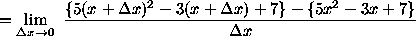(Algebraically and arithmetically simplify the expression in the numerator. Please note that there are TWO TYPOS in the numerator of the following quotient. The term "-3x^2+5x" should be "-5x^2+3x". However, steps thereafter are correct.)(The remaining steps are correct.)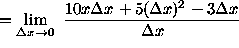(Factorfrom the expression in the numerator.)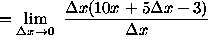(The termnow divides out and the limit can be calculated.)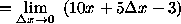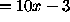.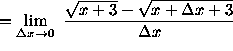(Eliminate the square root terms in the numerator of the expression by multiplying

by the conjugate of the numerator divided by itself.)(Recall that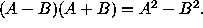)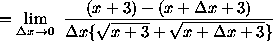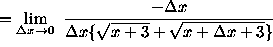(The termnow divides out and the limit can be calculated.)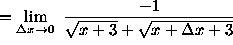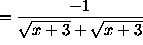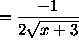.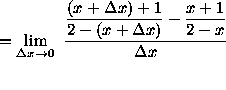(Get a common denominator for the expression in the numerator. Recall that division byis the same as multiplication by. )(Algebraically and arithmetically simplify the expression in the numerator. It is important to note that the denominator of this expression should be left in factored form so that the termcan be easily eliminated later.)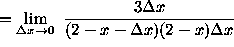(The termnow divides out and the limit can be calculated.).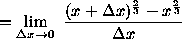(At this point it may appear that multiplying by the conjugate of the numerator over

itself is a good next step. However, doing something else is a better idea.)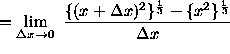(Note that A - B can be written as the difference of cubes , so that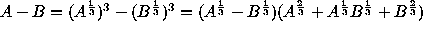. This will help explain the next step.)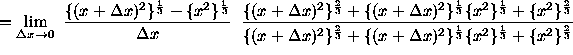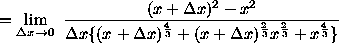(Algebraically and arithmetically simplify the expression in the numerator.)(The termnow divides out and the limit can be calculated.).(Recall a well-known trigonometry identity :.)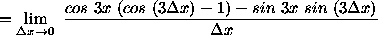(Recall the following two well-known trigonometry limits :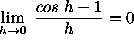and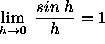.)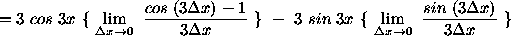.